# Mathematical Logic 2016 Propositional Logic exercises Fausto Giunchiglia

• Slides: 41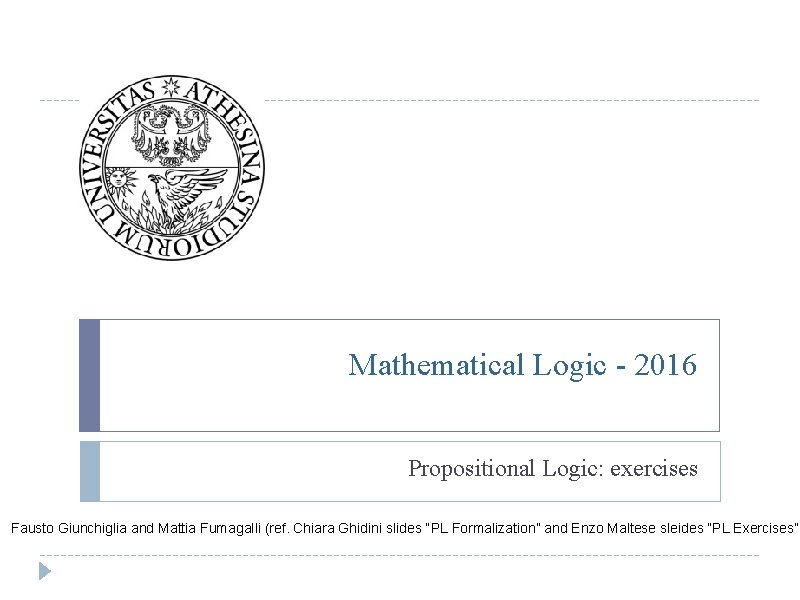Mathematical Logic - 2016 Propositional Logic: exercises Fausto Giunchiglia and Mattia Fumagalli (ref. Chiara Ghidini slides “PL Formalization” and Enzo Maltese sleides “PL Exercises”)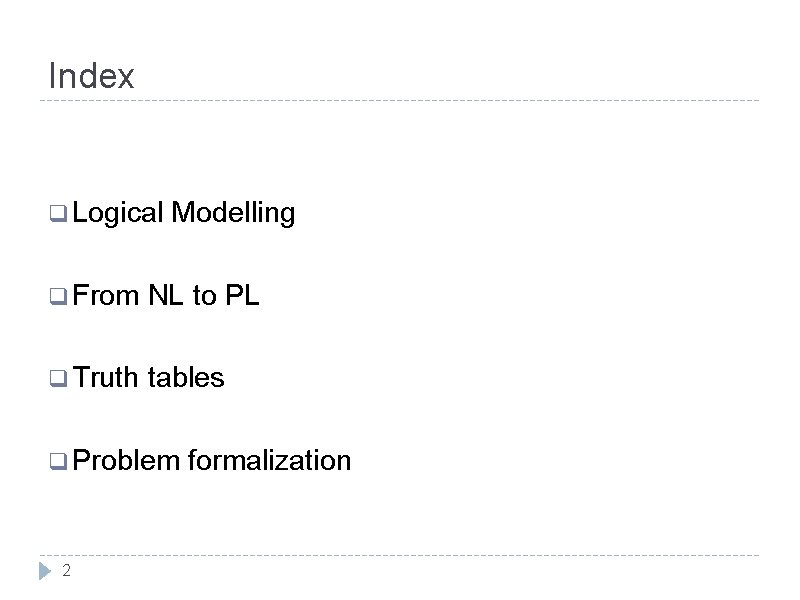Index q Logical Modelling q From NL to PL q Truth tables q Problem formalization 2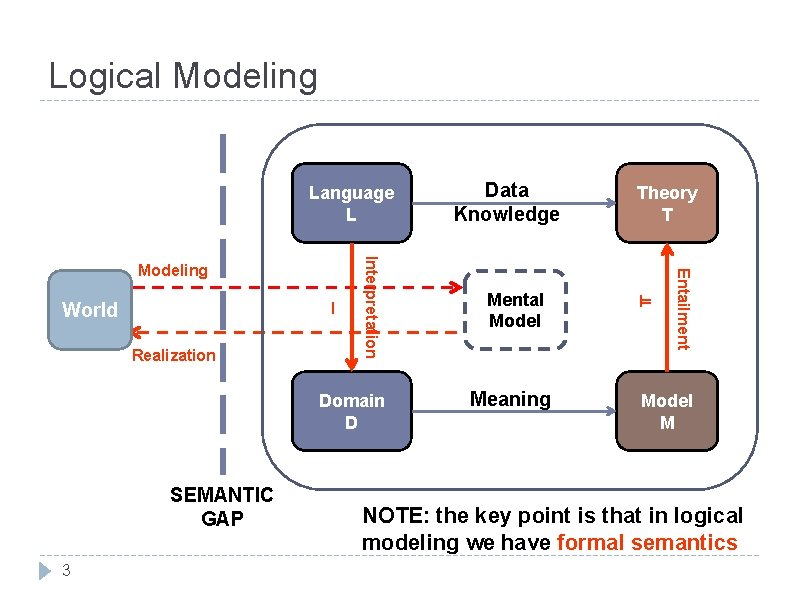Logical Modeling Language L Realization Domain D SEMANTIC GAP 3 Mental Model Meaning Theory T ⊨ Entailment I World Interpretation Modeling Data Knowledge Model M NOTE: the key point is that in logical modeling we have formal semanticsMODELING : : LOGICAL MODELING : : INTENTIONAL MODELS : : EXTENSIONAL MODELS Modeling Exercise: Forest q Description: There are two lions, Kimba and Simba, in the forest. They are in competition for the food. There is a nice antelope they want to hunt. If they want to survive they have to catch it. q Problem: Model the problem by identify relevant objects, defining the domain, the language, theory and providing a possible intentional model. 4Solution: Forest (I) q Description: There are two lions, Kimba and Simba, in the forest. They are in competition for the food. There is a nice antelope they want to hunt. If they want to survive they have to catch it. Relevant objects are in red D = {T, F} L = {Lion, Antelope, Survive, Catch} 5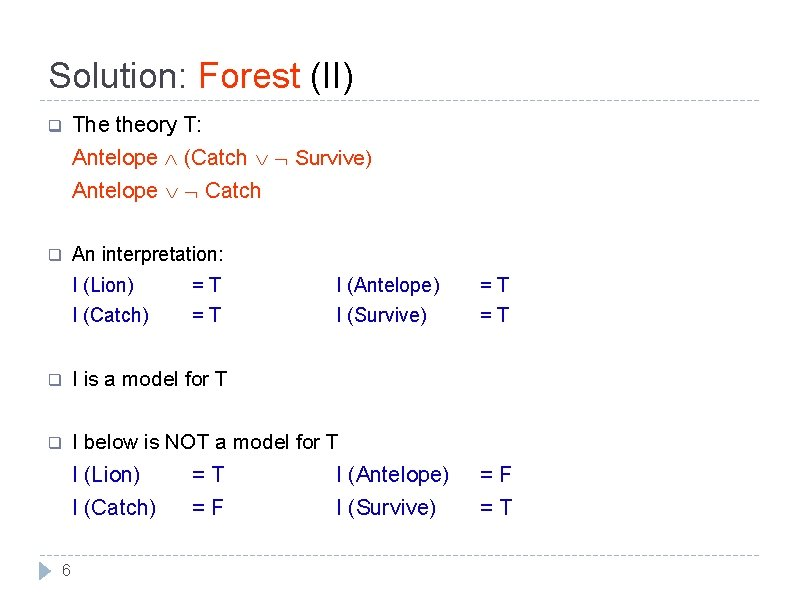Solution: Forest (II) q The theory T: Antelope (Catch Survive) Antelope Catch q An interpretation: I (Lion) = T I (Antelope) = T I (Catch) = T I (Survive) = T q I is a model for T q I below is NOT a model for T 6 I (Lion) = T I (Antelope) = F I (Catch) = F I (Survive) = T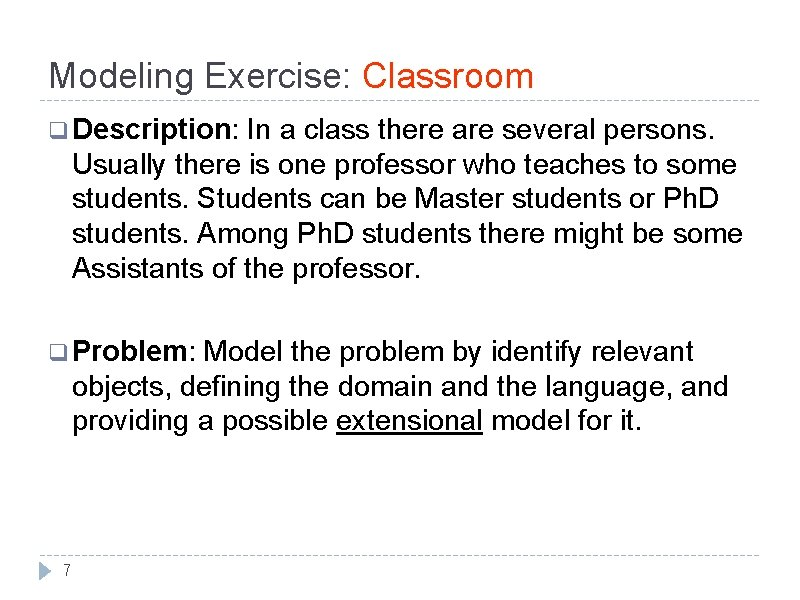Modeling Exercise: Classroom q Description: In a class there are several persons. Usually there is one professor who teaches to some students. Students can be Master students or Ph. D students. Among Ph. D students there might be some Assistants of the professor. q Problem: Model the problem by identify relevant objects, defining the domain and the language, and providing a possible extensional model for it. 7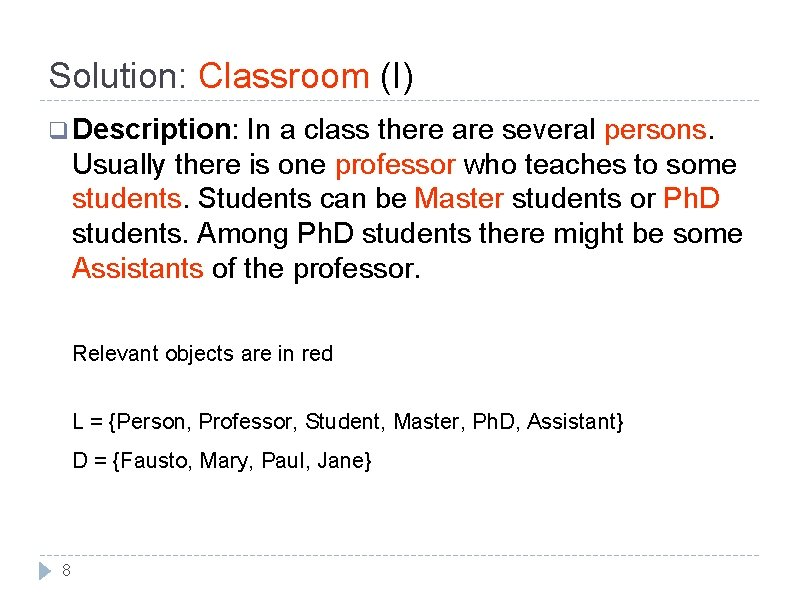Solution: Classroom (I) q Description: In a class there are several persons. Usually there is one professor who teaches to some students. Students can be Master students or Ph. D students. Among Ph. D students there might be some Assistants of the professor. Relevant objects are in red L = {Person, Professor, Student, Master, Ph. D, Assistant} D = {Fausto, Mary, Paul, Jane} 8Solution: Classroom (II) q The corresponding Venn diagram U Ph. D Assistant Student Master 9 Professor PersonSolution: Classroom (III) q A possible model: I (Person) = {Fausto, Mary, Paul, Jane} I (Professor) = {Fausto} I (Student) = {Mary, Paul, Jane} I (Master) = {Mary} I (Ph. D) = {Paul, Jane} I (Assistant) = {Paul} 10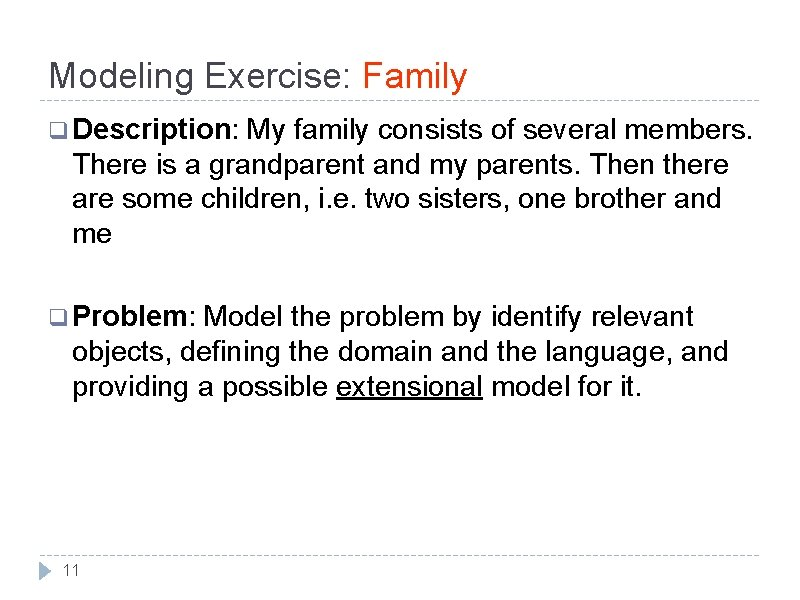Modeling Exercise: Family q Description: My family consists of several members. There is a grandparent and my parents. Then there are some children, i. e. two sisters, one brother and me q Problem: Model the problem by identify relevant objects, defining the domain and the language, and providing a possible extensional model for it. 11Solution: Family (I) q Description: My family consists of several members. There is a grandparent and my parents. Then there are some children, i. e. two sisters, one brother and me Relevant objects are in red L = {member, grandparent, child, brother, sister, me} D = {Bob, Fausto, Mary, Paul, Jane, Hugo, Robert} 12Solution: Family (II) q The corresponding Venn diagram U Grandparent Parent Member Brother Child 13 Sister MeSolution: Family (III) q A possible model: I (Member) = {Bob, Fausto, Mary, Paul, Jane, Hugo, Robert} I (Grandparent) = {Bob} I (Parent) = {Fausto, Mary, Bob} I (Brother) = {Robert, Paul} I (Sister) = {Jane} I (Me) = {Hugo} 14Modeling Exercise: My friends q Description: I have a lot of friends. I met some of them on the forum of my website. However, only a few of them are close to me. In particular, I use to play chess with Paul. q Problem: Model the problem by identify relevant objects, defining the domain and the language, and providing a possible extensional model for it. 15Propositional logic language Propositional alphabet: q. Logical symbols: , ∧, ∨, →, and ↔ q. Non logical symbols A set Ω of symbols called propositional variables q. Separator symbols “(” and “)” q“Meta-symbols”, i. e. ⊨, ⊤ or ⊥ Definition (Well formed formulas): q. Every P ∈ Ω is an atomic formula q. Every atomic formula is a formula q. If A and B are formulas then A, A ∧ B, A ∨ B, A → B, e A ↔ B are formulas 16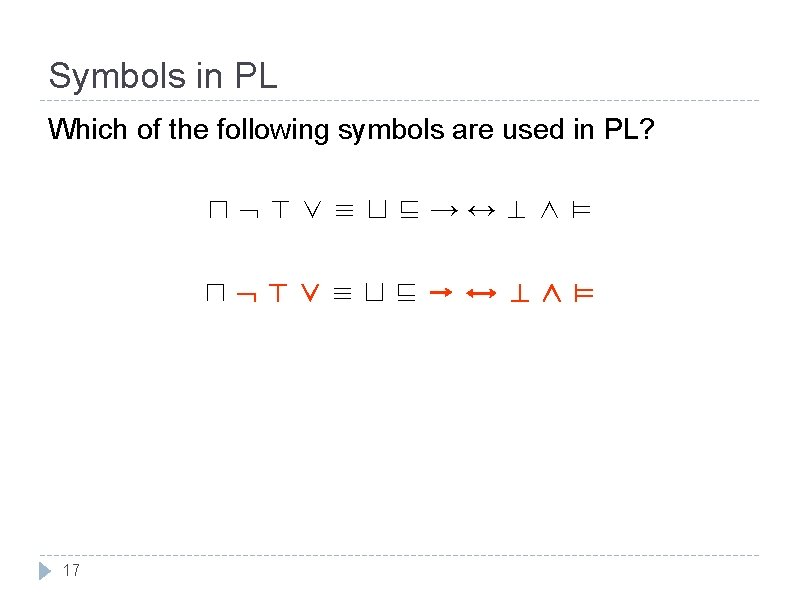Symbols in PL Which of the following symbols are used in PL? ⊓ ⊤ ∨ ≡ ⊔ ⊑ → ↔ ⊥ ∧ ⊨ ⊓ ⊤∨≡⊔⊑→↔⊥∧⊨ 17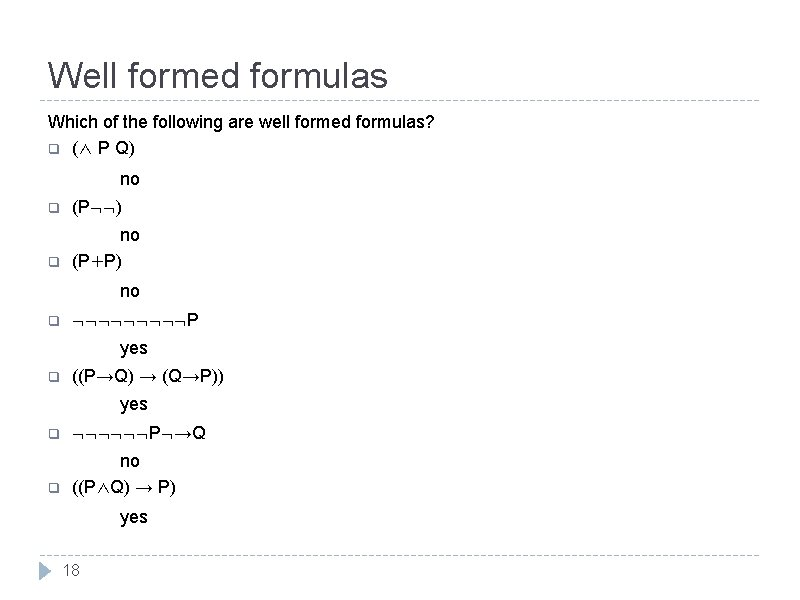Well formed formulas Which of the following are well formed formulas? q (∧ P Q) no q (P ) q no (P+P) no q P yes q ((P→Q) → (Q→P)) yes q P →Q q no ((P∧Q) → P) yes 18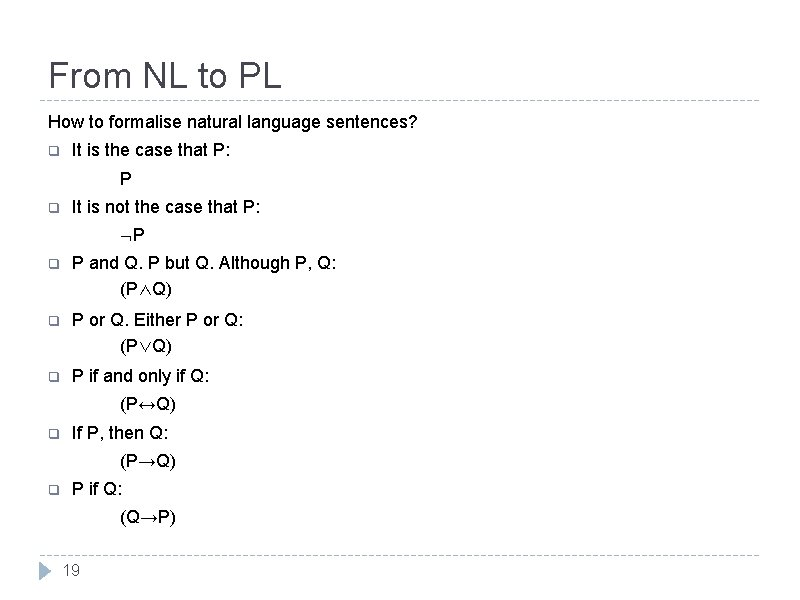From NL to PL How to formalise natural language sentences? q It is the case that P: P q It is not the case that P: P q P and Q. P but Q. Although P, Q: (P∧Q) q P or Q. Either P or Q: (P∨Q) q P if and only if Q: (P↔Q) q If P, then Q: (P→Q) q P if Q: (Q→P) 19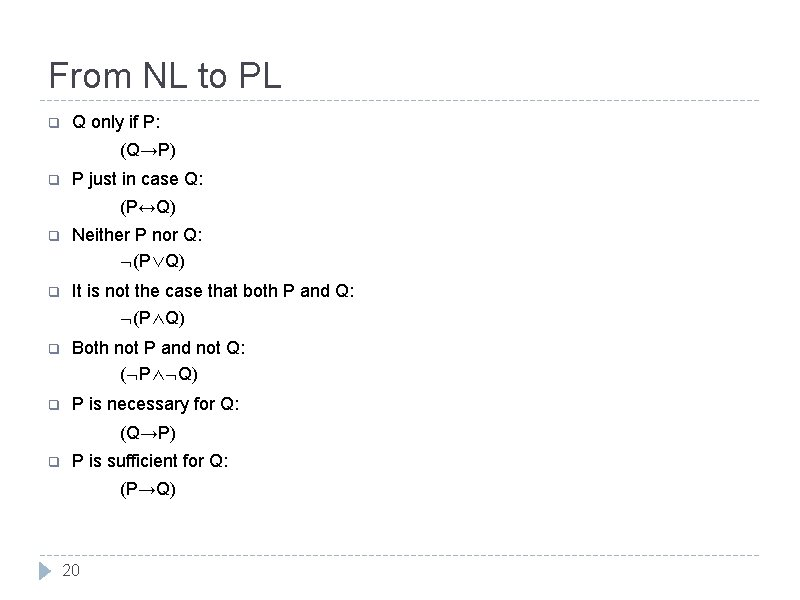From NL to PL q Q only if P: (Q→P) q P just in case Q: (P↔Q) q Neither P nor Q: (P∨Q) q It is not the case that both P and Q: (P∧Q) q Both not P and not Q: ( P∧ Q) q P is necessary for Q: (Q→P) q P is sufficient for Q: (P→Q) 20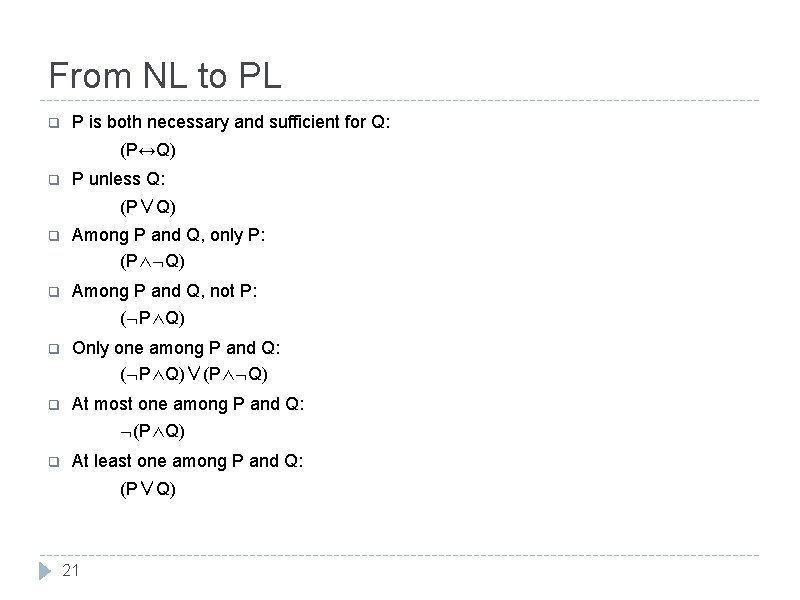From NL to PL q P is both necessary and sufficient for Q: (P↔Q) q P unless Q: (P∨Q) q Among P and Q, only P: (P∧ Q) q Among P and Q, not P: ( P∧Q) q Only one among P and Q: ( P∧Q)∨(P∧ Q) q At most one among P and Q: (P∧Q) q At least one among P and Q: (P∨Q) 21Formalizing NL Let's consider a propositional language where p means "Paola is happy", q means "Paola paints a picture", and r means "Renzo is happy". Formalize the following sentences: q"if Paola is happy and paints a picture then Renzo isn't happy” (p∧q) → r q"if Paola is happy, then she paints a picture” p → q q"Paola is happy only if she paints a picture” (p∧ q) which is equivalent to p → q !!! 22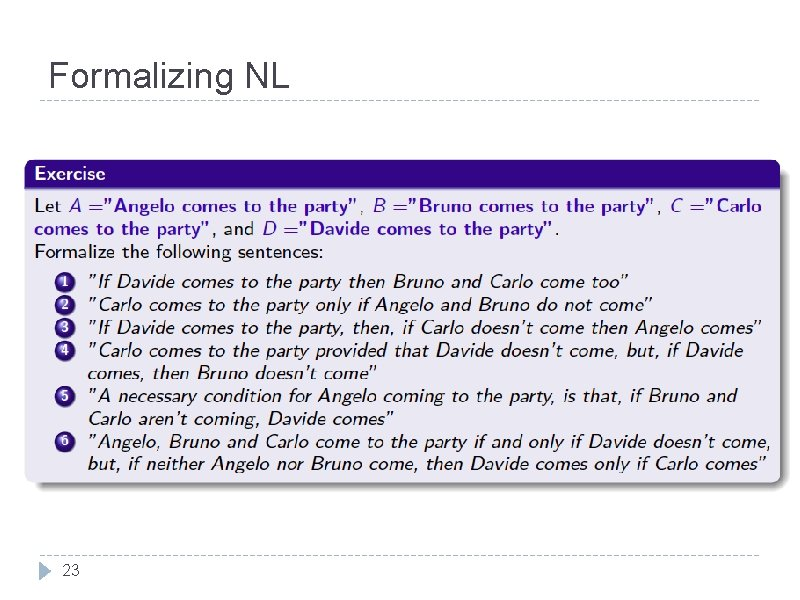Formalizing NL 23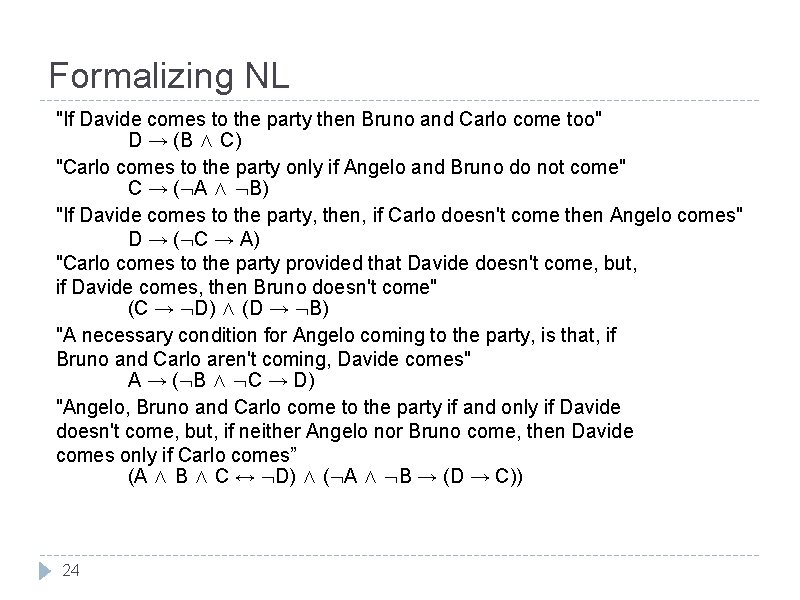Formalizing NL "If Davide comes to the party then Bruno and Carlo come too" D → (B ∧ C) "Carlo comes to the party only if Angelo and Bruno do not come" C → ( A ∧ B) "If Davide comes to the party, then, if Carlo doesn't come then Angelo comes" D → ( C → A) "Carlo comes to the party provided that Davide doesn't come, but, if Davide comes, then Bruno doesn't come" (C → D) ∧ (D → B) "A necessary condition for Angelo coming to the party, is that, if Bruno and Carlo aren't coming, Davide comes" A → ( B ∧ C → D) "Angelo, Bruno and Carlo come to the party if and only if Davide doesn't come, but, if neither Angelo nor Bruno come, then Davide comes only if Carlo comes” (A ∧ B ∧ C ↔ D) ∧ ( A ∧ B → (D → C)) 24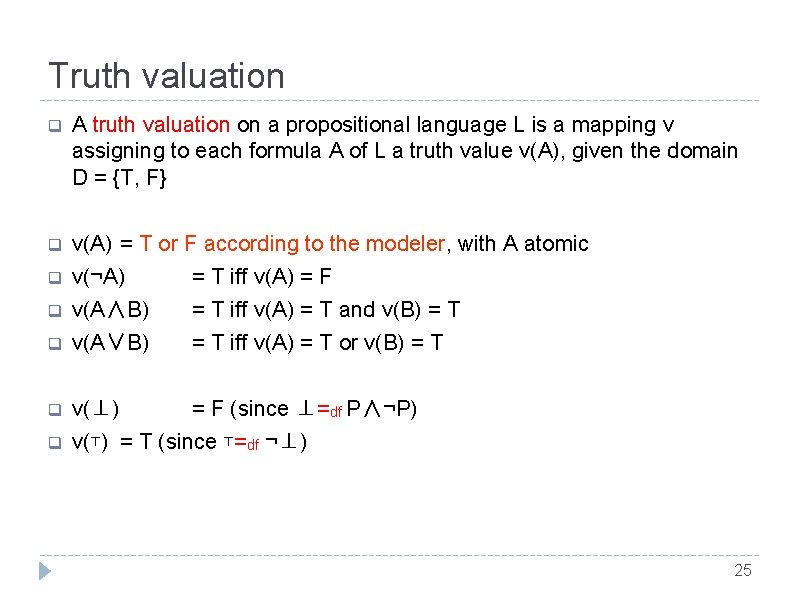Truth valuation q A truth valuation on a propositional language L is a mapping ν assigning to each formula A of L a truth value ν(A), given the domain D = {T, F} q ν(A) = T or F according to the modeler, with A atomic q ν(¬A) = T iff ν(A) = F q ν(A∧B) = T iff ν(A) = T and ν(B) = T q ν(A∨B) = T iff ν(A) = T or ν(B) = T q ν(⊥) = F (since ⊥=df P∧¬P) q ν(⊤) = T (since ⊤=df ¬⊥) 25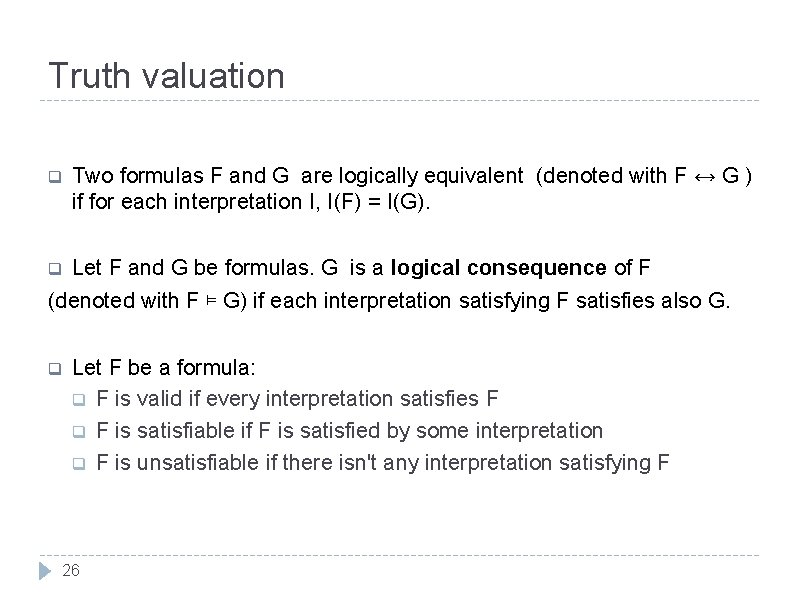Truth valuation q Two formulas F and G are logically equivalent (denoted with F ↔ G ) if for each interpretation I, I(F) = I(G). q Let F and G be formulas. G is a logical consequence of F (denoted with F ⊨ G) if each interpretation satisfying F satisfies also G. q Let F be a formula: q F is valid if every interpretation satisfies F q F is satisfiable if F is satisfied by some interpretation q F is unsatisfiable if there isn't any interpretation satisfying F 26Truth valuation and Truth Tables q A truth valuation on a PL language L is a mapping ν that assigns to each formula P of L a truth value ν(P). q A truth table is composed of one column for each input variable and one (or more) final column for all of the possible results of the logical operation that the table is meant to represent. Each row of the truth table therefore contains one possible assignment of the input variables, and the result of the operation for those values. LOGICAL OPERATION VARIABLES POSSIBLE ASSIGNEMENTS 27 A B A∧B T T F F F T F FExample q Calculate the Truth Table of the following formulas: (1) A ∧ B; (2) A ∨ B; (3) A ↔ B. VARIABLES POSSIBLE ASSIGNEMENTS 28 (1) (2) (3) A B A∧B A∨B A↔B T T T F F T F T F F F T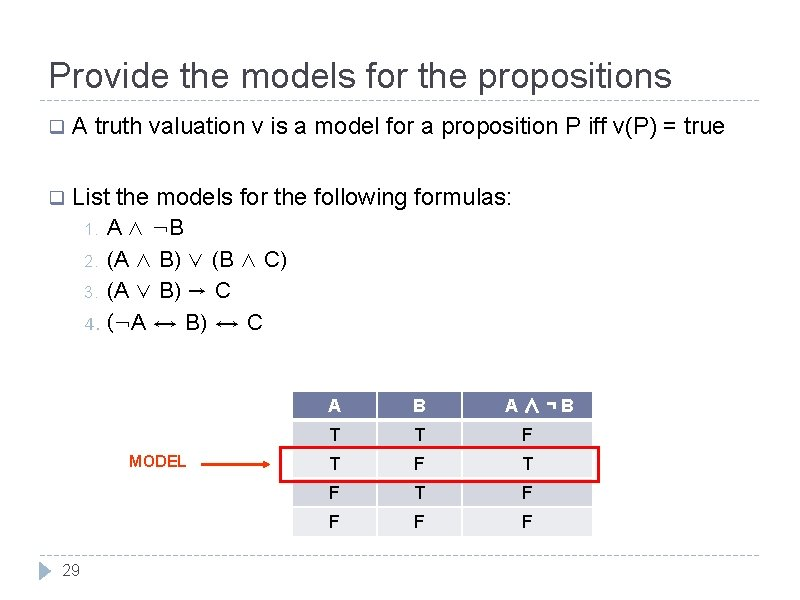Provide the models for the propositions q A truth valuation ν is a model for a proposition P iff ν(P) = true q List the models for the following formulas: A ∧ ¬B 2. (A ∧ B) ∨ (B ∧ C) 3. (A ∨ B) → C 4. (¬A ↔ B) ↔ C 1. MODEL 29 A B A∧¬B T T F T F F F FTruth Tables Example (1) Use the truth tables method to determine whether (p → q) ∨ (p → q) is valid. The formula is valid since it is satisfied by every interpretation. 30Truth Tables Example (2) Use the truth tables method to determine whether ( p ∨ q) ∧ (q → r ∧ p) ∧ (p ∨ r) (denoted with F) is valid. There exists an interpretation satisfying F, thus F is satisfiable. 31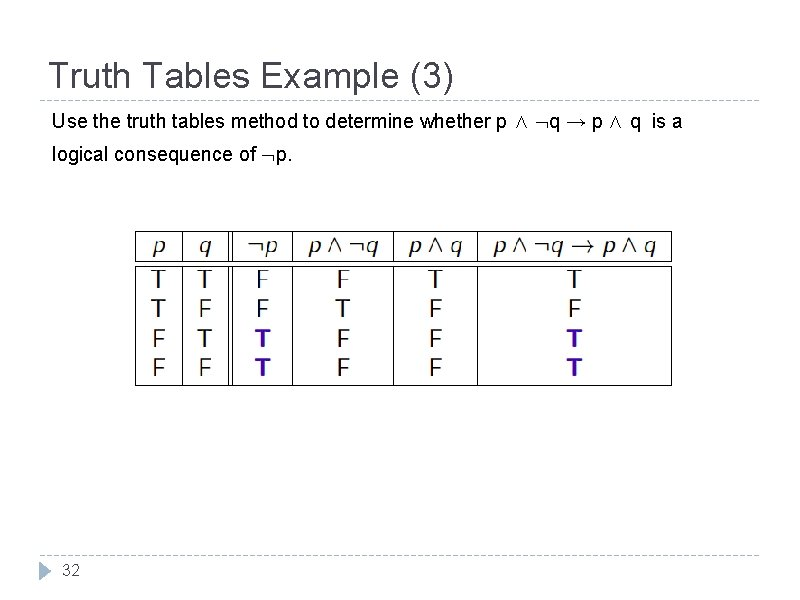Truth Tables Example (3) Use the truth tables method to determine whether p ∧ q → p ∧ q is a logical consequence of p. 32Truth Tables Example (4) Use the truth tables method to determine whether p → (q ∧ q) and p are logically equivalent. 33Truth Tables Exercises 34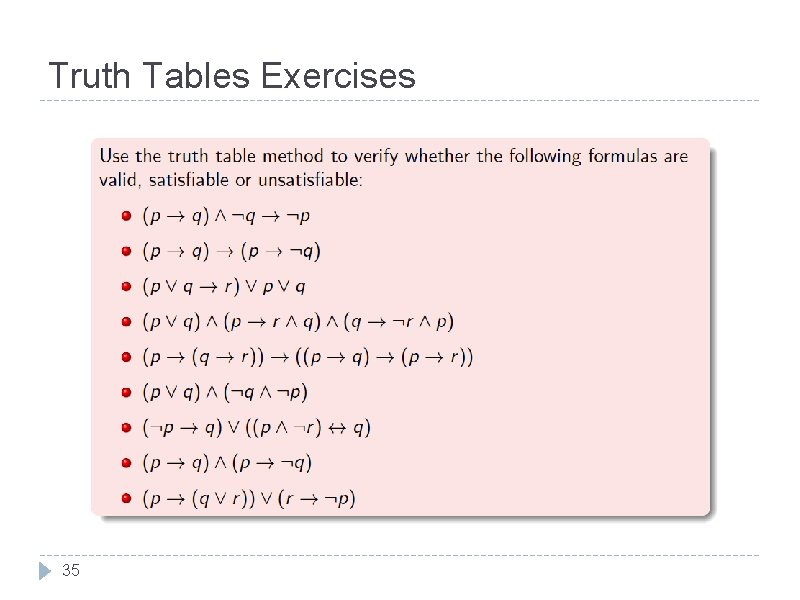Truth Tables Exercises 35Problem formalization (1) Formalize the following arguments and verify whether they are correct: "If you play and you study you'll pass the exams, while if you play and don't study you won't pass. Thus, if you play, either you study and you'll pass the exams, or you don't study and you won't pass. ” L = {P, S, E} P = “you play”, S = “you study”; E = “you pass the exam” 1. (P ∧ S) E 2. (P ∧ S) E 3. P (S ∧ E) ( S ∧ E) We need to prove that 1 ∧ 2 ⊨ 3 Use truth tables 36Problem formalization (2) Brown, Jones, and Smith are suspected of a crime. They testify as follows: Brown: “Jones is guilty and Smith is innocent”. Jones: “If Brown is guilty then so is Smith”. Smith: “I'm innocent, but at least one of the others is guilty”. Let B, J, and S be the statements “Brown is guilty”, “Jones is guilty”, and “Smith is guilty”, respectively. Do the following: 1. Express the testimony of each suspect as a PL formula. The three statements can be expressed as J ∧ S, B S, and S ∧ (B ∨ J) 37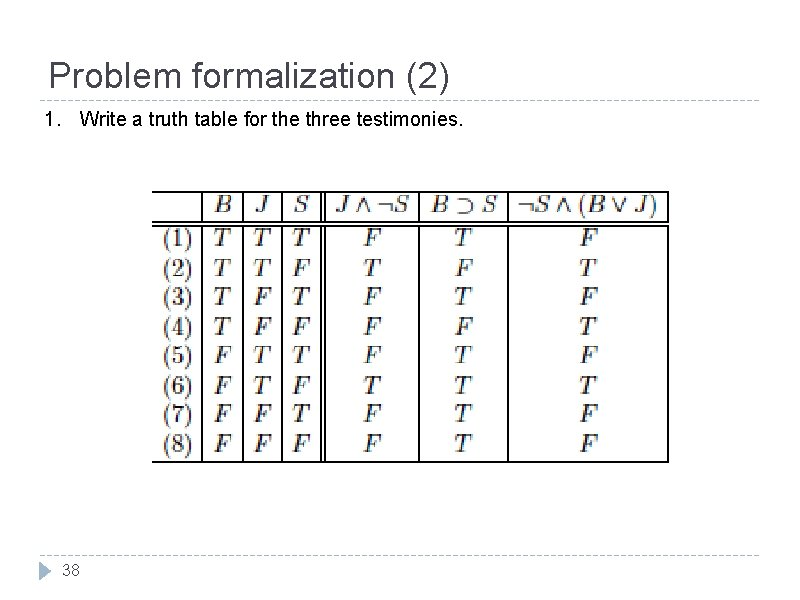Problem formalization (2) 1. Write a truth table for the three testimonies. 38Problem formalization (2) Use the truth table to answer the following questions: (a) Are three testimonies satisfiable? Yes, assignment (6) makes them all true (b) The testimony of one of the suspects follows from that of another. Which from which? J ∧ S ⊨ S ∧ (B ∨ J) (c) Assuming that everybody is innocent, who committed perjury? Everybody is innocent corresponds to assignment (8), and in this case the statements of Brown and Smith are false. (d) Assuming that all testimonies are true, who is innocent and who is guilty? Assuming that all testimonies are true corresponds to assignment (6). In this case Jones is guilty and the others are innocents. 39Problem formalization (3) 40Problem formalization (3) r : "freedom is behind the red door" b: "freedom is behind the blue door" g: "freedom is behind the green door" "behind one of the door is a path to freedom, behind the other two doors is an evil dragon" (r ∧ b ∧ g) ∨ ( r ∧ b ∧ g) "at least one of the three statements is true" r ∨ b "at least one of the three statements is false" r ∨ b Freedom is behind the green door! 41